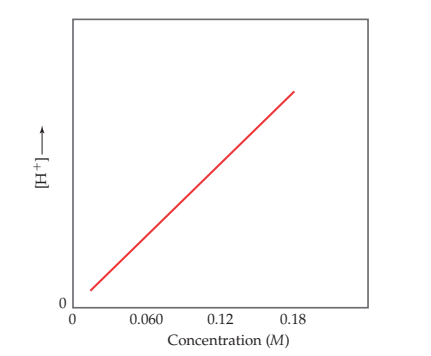×
Get Full Access to Chemistry: The Central Science - 14 Edition - Chapter 16 - Problem 16.6
Get Full Access to Chemistry: The Central Science - 14 Edition - Chapter 16 - Problem 16.6

×

# ?The graph given below shows $$\left[\mathrm{H}^{+}\right]$$ versus concentration for an aqueous solution of an unknown substance. (a) Is theISBN: 9780134414232 1274

## Solution for problem 16.6 Chapter 16

Chemistry: The Central Science | 14th Edition

• Textbook Solutions
• 2901 Step-by-step solutions solved by professors and subject experts
• Get 24/7 help from StudySoup virtual teaching assistantsChemistry: The Central Science | 14th Edition

4 5 1 420 Reviews
11
2
Problem 16.6

The graph given below shows $$\left[\mathrm{H}^{+}\right]$$ versus concentration for an aqueous solution of an unknown substance.

(a) Is the substance a strong acid, a weak acid, a strong base, or a weak base?

(b) Based on your answer to (a), can you determine the value of the pH of the solution when the concentration is 0.18 M?

(c) Would the line go exactly through the origin of the plot? [Sections 16.5 and 16.6]Text Transcription:

[H+]

Step-by-Step Solution:

Step 1 of 5) Any living organism is a complex, highly organized, well-ordered system, even at the molecular level like the nucleosome we saw at the beginning of this chapter. Our entropy content is much lower than it would be if we were completely decomposed into carbon dioxide, water, and several other simple chemicals. Does this mean that life is a violation of the second law No, because the thousands of chemical reactions necessary to produce and maintain life have caused a large increase in the entropy of the rest of the universe. Thus, as the second law requires, the overall entropy change during the lifetime of a human, or any other living system, is positive. The second law of thermodynamics applies also to the way we humans order our surroundings. In addition to being complex living systems ourselves, we are masters of producing order in the world around us.

Step 2 of 2

## Discover and learn what students are asking

Calculus: Early Transcendental Functions : Exponential and Logarithmic Functions
?In Exercises 7-22, solve for $$x$$. $$3^{x}=81$$

Calculus: Early Transcendental Functions : Partial Derivatives
?In Exercises 7 - 38, find both first partial derivatives. $$z=y e^{y / x}$$

Calculus: Early Transcendental Functions : Iterated Integrals and Area in the Plane
?In Exercises 1 - 10, evaluate the integral. $$\int_{1}^{2 y} \frac{y}{x} d x, \quad y>0$$

Statistics: Informed Decisions Using Data : Applications of the Normal Distribution
?Explain why P(X ? 220) should be reported as > 70.9999 if X is a normal random variable with mean 100 and standard deviation 15.

Unlock Textbook Solution

?The graph given below shows $$\left[\mathrm{H}^{+}\right]$$ versus concentration for an aqueous solution of an unknown substance. (a) Is the